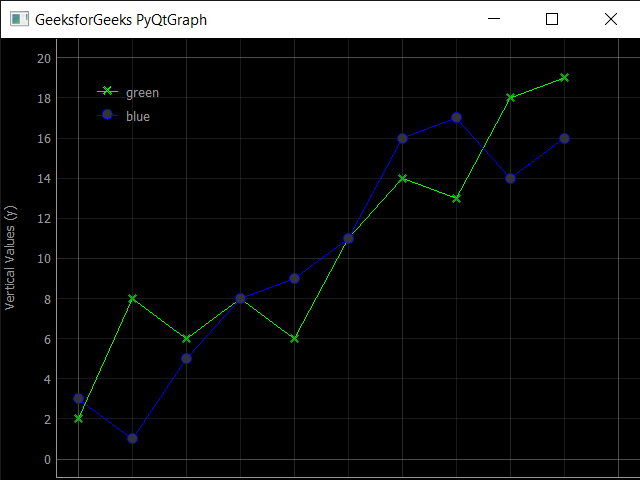PyQtGraph – Plot Window

• Last Updated : 06 Sep, 2021

In this article we will see how we can create plot window in the PyQtGraph module. PyQtGraph is a graphics and user interface library for Python that provides functionality commonly required in designing and science applications. Its primary goals are to provide fast, interactive graphics for displaying data (plots, video, etc.) and second is to provide tools to aid in rapid application development (for example, property trees such as used in Qt Designer).
Plot windows consist of two main parts: the Plot Panel containing the actual plotted graphics (by default, at the top), and the Control Panel (by default, at the bottom). The Control Panel is where you configure what will be plotted. For a simple scatter plot it may just be a case of selecting what columns to plot against each other, but it can get quite detailed.

In order to do this we use plot method with the pyqtgraph
Syntax : pg.plot()
Argument : It takes no argument
Return : It returns PlotWindow object

Below is the implementation

Python3

 # importing pyqtgraph as pgimport pyqtgraph as pg # importing QtCore and QtGui from the pyqtgraph modulefrom pyqtgraph.Qt import QtCore, QtGui # importing numpy as npimport numpy as np # define the datatitle = "GeeksforGeeks PyQtGraph" # y values to plot by line 1y = [2, 8, 6, 8, 6, 11, 14, 13, 18, 19] # y values to plot by line 2y2 = [3, 1, 5, 8, 9, 11, 16, 17, 14, 16]x = range(0, 10) # create plot window objectplt = pg.plot() # showing x and y gridsplt.showGrid(x = True, y = True) # adding legendplt.addLegend() # set properties of the label for y axisplt.setLabel('left', 'Vertical Values', units ='y') # set properties of the label for x axisplt.setLabel('bottom', 'Horizontal Values', units ='s') # setting horizontal rangeplt.setXRange(0, 10) # setting vertical rangeplt.setYRange(0, 20) # setting window titleplt.setWindowTitle(title) # ploting line in green colorline1 = plt.plot(x, y, pen ='g', symbol ='x', symbolPen ='g',                           symbolBrush = 0.2, name ='green') # ploting line2 with blue colorline2 = plt.plot(x, y2, pen ='b', symbol ='o', symbolPen ='b',                             symbolBrush = 0.2, name ='blue')   # main methodif __name__ == '__main__':         # importing system    import sys         # Start Qt event loop unless running in interactive mode or using    if (sys.flags.interactive != 1) or not hasattr(QtCore, 'PYQT_VERSION'):        QtGui.QApplication.instance().exec_()

Output :My Personal Notes arrow_drop_up